Monday, 26 March 2018 08:29

### Add a Cover Image when Converting Word to EPUB in C#, VB.NET

We already have the documentation introducing how to convert Word to EPUB. However, you may want to add a cover image to EPUB when creating an EPUB book from a Word document. The following code snippets will demonstrate the same.

Step 1: Create a Document instance and load a sample Word file.

```Document doc = new Document();
```

Step 2: Load a picture to DocPicture object.

```DocPicture picture = new DocPicture(doc);
```

Step 3: Add the picture to EPUB as cover image when creating EPUB from the Word document.

```doc.SaveToEpub("output.epub", picture);
```

Output: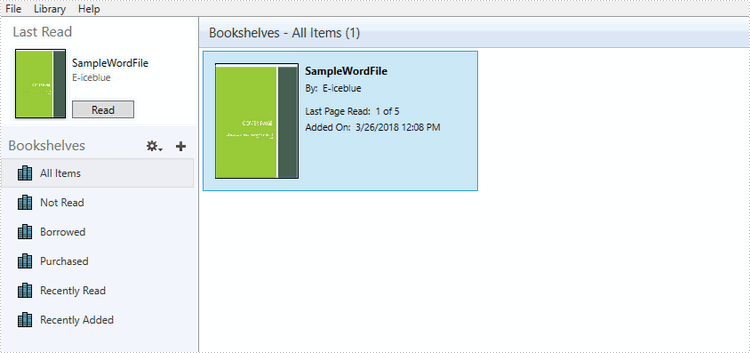Full Code:

[C#]
```Document doc = new Document();

DocPicture picture = new DocPicture(doc);
doc.SaveToEpub("output.epub", picture);
```
[VB.NET]
```Document doc  =  New Document()

Dim picture As DocPicture =  New DocPicture(doc)
doc.SaveToEpub("output.epub", picture)
```
Thursday, 22 March 2018 08:29

### Create 100% stacked bar chart in PowerPoint in C#

A 100% stacked bar chart is a chart type designed to show the relative percentage of multiple data series in stacked bars, where the total of each stacked bar always equals 100%. This article will demonstrate how to use Spire.Presentation to create 100% stacked bar in PowerPoint in C#.

Step 1: Create a Presentation object.

```Presentation presentation = new Presentation();
```

Step 2: Add a "Bar100PercentStacked" chart to the first slide.

```presentation.SlideSize.Type = SlideSizeType.Screen16x9;
SizeF slidesize = presentation.SlideSize.Size;
var slide = presentation.Slides;

RectangleF rect = new RectangleF(20, 20, slidesize.Width - 40, slidesize.Height - 40);
IChart chart = slide.Shapes.AppendChart(Spire.Presentation.Charts.ChartType.Bar100PercentStacked, rect);
```

Step 3: Write data to the chart data.

```string[] columnlabels = { "Series 1", "Series 2", "Series 3" };

// Insert the column labels
String[] cols = columnlabels.ToArray();
for (Int32 c = 0; c < cols.Count(); ++c)
chart.ChartData[0, c + 1].Text = cols[c];

string[] rowlabels = { "Category 1", "Category 2", "Category 3" };

// Insert the row labels
String[] rows = rowlabels.ToArray();
for (Int32 r = 0; r < rows.Count(); ++r)
chart.ChartData[r + 1, 0].Text = rows[r];

double[,] values = new double[3, 3] { { 20.83233, 10.34323, -10.354667 }, { 10.23456, -12.23456, 23.34456 }, { 12.34345, -23.34343, -13.23232 }};

// Insert the values
double value = 0.0;
for (Int32 r = 0; r < rows.Count(); ++r)
{
for (Int32 c = 0; c < cols.Count(); ++c)
{
value = Math.Round(values[r, c], 2);
chart.ChartData[r + 1, c + 1].Value = value;
}
}

chart.Series.SeriesLabel = chart.ChartData[0, 1, 0, columnlabels.Count()];
chart.Categories.CategoryLabels = chart.ChartData[1, 0, rowlabels.Count(), 0];

chart.PrimaryCategoryAxis.Position = AxisPositionType.Left;
chart.SecondaryCategoryAxis.Position = AxisPositionType.Left;
chart.PrimaryCategoryAxis.TickLabelPosition = TickLabelPositionType.TickLabelPositionLow;
```

Step 4: Set the data, font and format for the series of each column.

```for (Int32 c = 0; c < cols.Count(); ++c)
{
chart.Series[c].Values = chart.ChartData[1, c + 1, rowlabels.Count(), c + 1];
chart.Series[c].Fill.FillType = FillFormatType.Solid;
chart.Series[c].InvertIfNegative = false;

for (Int32 r = 0; r < rows.Count(); ++r)
{
label.LabelValueVisible = true;
chart.Series[c].DataLabels[r].HasDataSource = false;
chart.Series[c].DataLabels[r].NumberFormat = "0#\\%";
chart.Series[c].DataLabels.TextProperties.Paragraphs.DefaultCharacterProperties.FontHeight = 12;
}
}
chart.Series.Fill.SolidColor.Color = Color.YellowGreen;
chart.Series.Fill.SolidColor.Color = Color.Red;
chart.Series.Fill.SolidColor.Color = Color.Green;

TextFont font = new TextFont("Tw Cen MT");
```

Step 5: Set the font and size for chartlegend.

```for (int k = 0; k < chart.ChartLegend.EntryTextProperties.Length; k++)
{
chart.ChartLegend.EntryTextProperties[k].LatinFont = font;
chart.ChartLegend.EntryTextProperties[k].FontHeight = 20;
}
```

Step 6: Save the document to file.

```presentation.SaveToFile("Sample.pptx", FileFormat.Pptx2010);
```

Effective screenshot of 100% stacked bar chart: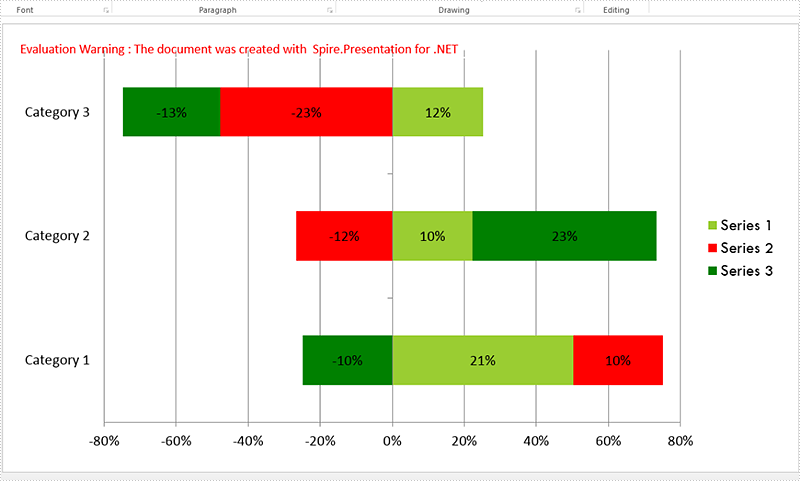Full codes:

```Presentation presentation = new Presentation();
presentation.SlideSize.Type = SlideSizeType.Screen16x9;
SizeF slidesize = presentation.SlideSize.Size;

var slide = presentation.Slides;

RectangleF rect = new RectangleF(20, 20, slidesize.Width - 40, slidesize.Height - 40);
IChart chart = slide.Shapes.AppendChart(Spire.Presentation.Charts.ChartType.Bar100PercentStacked, rect);

string[] columnlabels = { "Series 1", "Series 2", "Series 3" };

String[] cols = columnlabels.ToArray();
for (Int32 c = 0; c < cols.Count(); ++c)
chart.ChartData[0, c + 1].Text = cols[c];

string[] rowlabels = { "Category 1", "Category 2", "Category 3" };

String[] rows = rowlabels.ToArray();
for (Int32 r = 0; r < rows.Count(); ++r)
chart.ChartData[r + 1, 0].Text = rows[r];

double[,] values = new double[3, 3] { { 20.83233, 10.34323, -10.354667 }, { 10.23456, -12.23456, 23.34456 }, { 12.34345, -23.34343, -13.23232 }};

double value = 0.0;
for (Int32 r = 0; r < rows.Count(); ++r)
{
for (Int32 c = 0; c < cols.Count(); ++c)
{
value = Math.Round(values[r, c], 2);
chart.ChartData[r + 1, c + 1].Value = value;
}
}

chart.Series.SeriesLabel = chart.ChartData[0, 1, 0, columnlabels.Count()];
chart.Categories.CategoryLabels = chart.ChartData[1, 0, rowlabels.Count(), 0];

chart.PrimaryCategoryAxis.Position = AxisPositionType.Left;
chart.SecondaryCategoryAxis.Position = AxisPositionType.Left;
chart.PrimaryCategoryAxis.TickLabelPosition = TickLabelPositionType.TickLabelPositionLow;

for (Int32 c = 0; c < cols.Count(); ++c)
{
chart.Series[c].Values = chart.ChartData[1, c + 1, rowlabels.Count(), c + 1];
chart.Series[c].Fill.FillType = FillFormatType.Solid;
chart.Series[c].InvertIfNegative = false;

for (Int32 r = 0; r < rows.Count(); ++r)
{
label.LabelValueVisible = true;
chart.Series[c].DataLabels[r].HasDataSource = false;
chart.Series[c].DataLabels[r].NumberFormat = "0#\\%";
chart.Series[c].DataLabels.TextProperties.Paragraphs.DefaultCharacterProperties.FontHeight = 12;
}
}
chart.Series.Fill.SolidColor.Color = Color.YellowGreen;
chart.Series.Fill.SolidColor.Color = Color.Red;
chart.Series.Fill.SolidColor.Color = Color.Green;

TextFont font = new TextFont("Tw Cen MT");

for (int k = 0; k < chart.ChartLegend.EntryTextProperties.Length; k++)
{
chart.ChartLegend.EntryTextProperties[k].LatinFont = font;
chart.ChartLegend.EntryTextProperties[k].FontHeight = 20;
}

presentation.SaveToFile("Sample.pptx", FileFormat.Pptx2010);
```
Tuesday, 20 March 2018 09:35

### Print a PDF in Greyscale in C#

Below is the example PDF file we used for demonstration: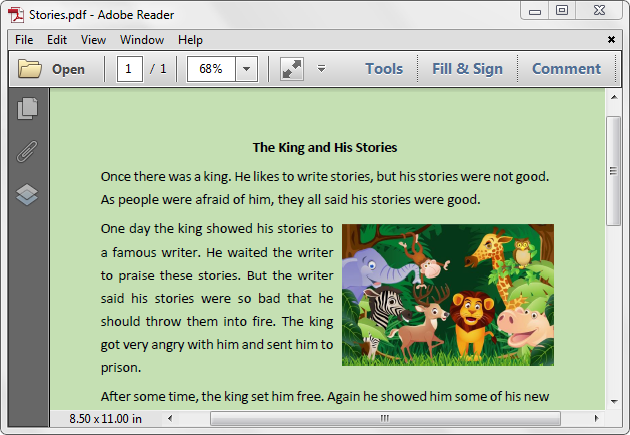Detail steps:

Step 1: Create a PdfDocument instance and load the PDF file.

```PdfDocument pdf = new PdfDocument();
```

Step 2: Set the PdfPrintSettings.Color property to false.

```pdf.PrintSettings.Color = false;
```

Step 3: Print the document.

```pdf.Print();
```

Screenshot after printing to xps: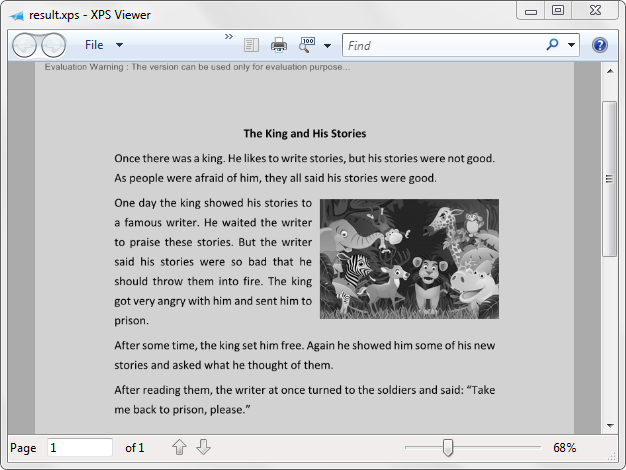Full code:

```using Spire.Pdf;

namespace Print_PDF_in_Black_and_White
{
class Program
{
static void Main(string[] args)
{
PdfDocument pdf = new PdfDocument();
pdf.PrintSettings.Color = false;
pdf.Print();
}
}
}
```
Monday, 19 March 2018 05:57

### Apply Shadow Effect to Shape in PowerPoint in C#

Shadows make your shapes or pictures especially with transparent background pop out of your slide. They make flat 2 dimensional graphics look like 3 dimensional graphics. This article will show you how we can apply shadow effects to shapes and pictures in PowerPoint using Spire.Presentation.

```//Create a Presentation object and get the first slide.
Presentation ppt = new Presentation();
ISlide slide = ppt.Slides;

RectangleF rect = new RectangleF(30, 80, 300, 120);
IAutoShape shape = slide.Shapes.AppendShape(ShapeType.Rectangle, rect);
shape.Fill.FillType = FillFormatType.Solid;
shape.Fill.SolidColor.Color = Color.LightBlue;
shape.Line.FillType = FillFormatType.None;
shape.TextFrame.Text = "This demo shows how to apply shadow effect to shape.";
shape.TextFrame.TextRange.Fill.FillType = FillFormatType.Solid;
shape.TextFrame.TextRange.Fill.SolidColor.Color = Color.Black;

//Apply the shadow effect to shape.

//Save to file.
```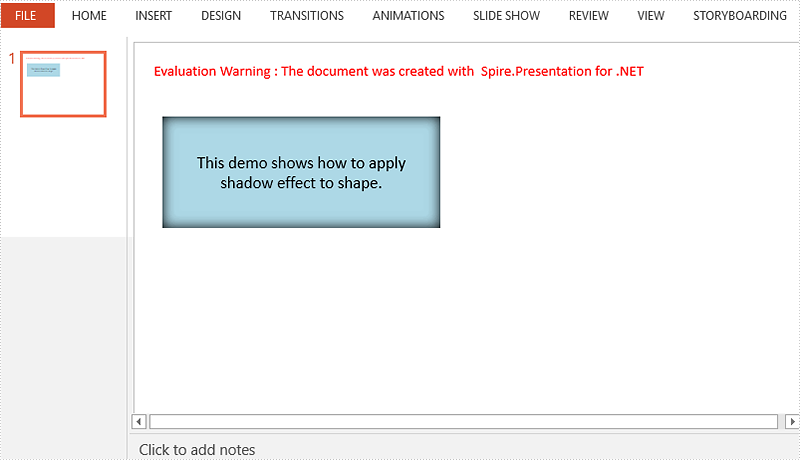```//Create a Presentation object and get the first slide.
Presentation ppt = new Presentation();
ISlide slide = ppt.Slides;

//Get the picture path.
string imagePath = "dinosaur.png";
Image image = Image.FromFile(imagePath);
float width = (float)image.Width / 3;
float height = (float)image.Height / 3;

//Add a shape to slide and fill the shape with picture.
RectangleF rect = new RectangleF(80, 80, width, height);
IAutoShape shape = slide.Shapes.AppendShape(ShapeType.Rectangle, rect);
shape.Fill.FillType = FillFormatType.Picture;
shape.Fill.PictureFill.Picture.Url = imagePath;
shape.Fill.PictureFill.FillType = PictureFillType.Stretch;
shape.Line.FillType = FillFormatType.None;

//Apply the shadow effect to shape.

//Save to file.
```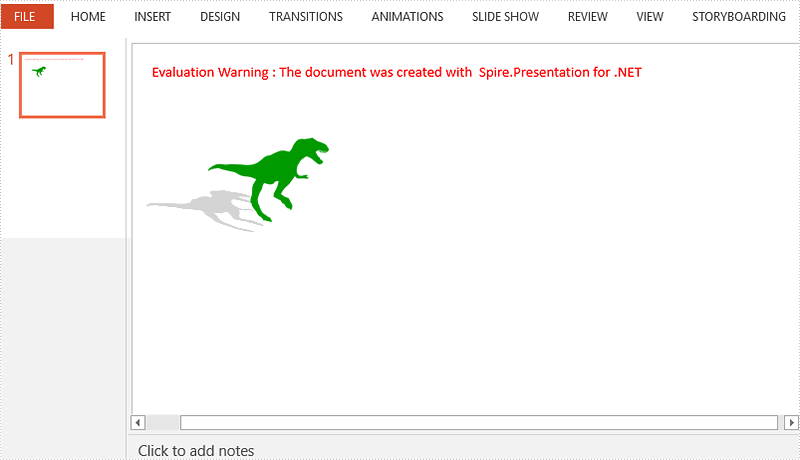Tuesday, 13 March 2018 08:11

### Convert the PDF to word, HTML, SVG, XPS and save them to stream

This article we will demonstrate how to convert the PDF pages to HTML, Word, SVG, XPS, PDF and save them to stream by calling the method PdfDocument.SaveToStream() offered by Spire.PDF. And starts from Spire.PDF version 4.3, it newly supports to convert the defined range of PDF pages and save them to stream.

#### Save the PDF to stream

Step 1: Create a new PdfDocument instance and load the sample document from file.

```PdfDocument pdf = new PdfDocument();
```

Step 2: Save the document to stream.

```MemoryStream ms=new MemoryStream ();
pdf.SaveToStream(ms);
```

#### Save the PDF to stream and defined the file format to HTML, Word, SVG, XPS and PDF

Step 1: Create a new PdfDocument instance and load the sample document from file.

```PdfDocument pdf = new PdfDocument();
```

Step 2: Save the document to stream and use FileFormat format to define the format.

```MemoryStream ms=new MemoryStream ();
pdf.SaveToStream(ms, FileFormat.HTML);
```

#### Convert the defined range of PDF pages to HTML, word, SVG, XPS and save them to stream

Step 1: Create a new PdfDocument instance and load the sample document from file.

```PdfDocument pdf = new PdfDocument();
```

Step 2: Only save some PDF pages to stream by using pdf.SaveToStream(int startIndex, int endIndex, FileFormat format) method; and FileFormat.PDF is not supported.

```pdf.SaveToStream(1, 2, FileFormat.SVG);
```

Full codes of save PDF to stream:

```PdfDocument pdf = new PdfDocument();

MemoryStream ms=new MemoryStream ();
pdf.SaveToStream(ms);
pdf.SaveToStream(ms, FileFormat.HTML);

pdf.SaveToStream(1, 2, FileFormat.SVG);
```
Monday, 12 March 2018 07:55

### Delete Legend and Specific Legend Entries from Excel Chart in C#

A legend is displayed in the chart area by default. However it can be removed from the chart. With Spire.XLS, we can delete the whole legend as well as specific legend entries from Excel chart. This article is going to demonstrate how we can use Spire.XLS to accomplish this function.

Below screenshot shows the Excel chart we used for demonstration: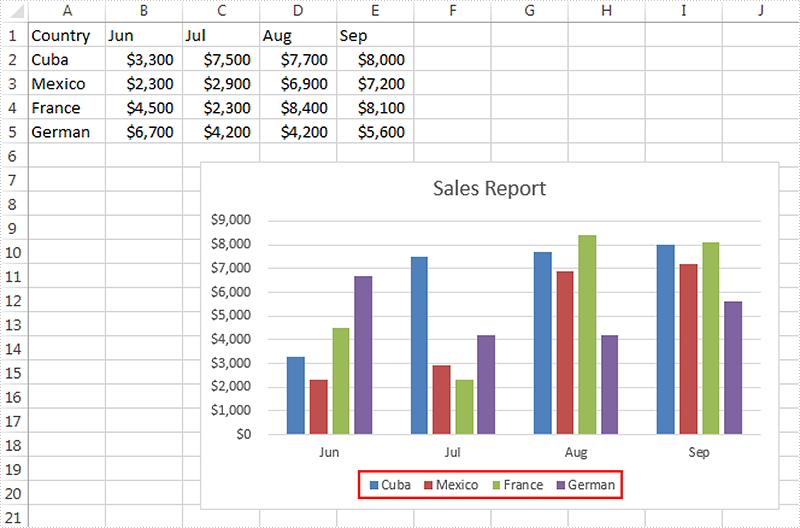Delete the whole legend

```//Create a Workbook instance
Workbook workbook = new Workbook();

//Get the first worksheet
Worksheet sheet = workbook.Worksheets;

//Get the chart
Chart chart = sheet.Charts;

//Delete legend from the chart
chart.Legend.Delete();

//Save the file
workbook.SaveToFile("DeleteLegend.xlsx", ExcelVersion.Version2013);
```

Screenshot: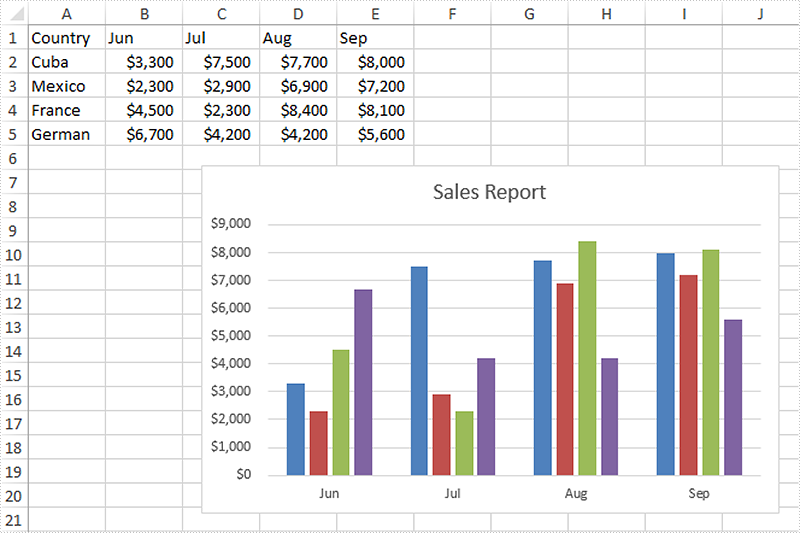Delete specific legend entries

```//Create a Workbook instance
Workbook workbook = new Workbook();

//Get the first worksheet
Worksheet sheet = workbook.Worksheets;

//Get the chart
Chart chart = sheet.Charts;

//Delete the first and the second legend entries from the chart
chart.Legend.LegendEntries.Delete();
chart.Legend.LegendEntries.Delete();

//Save the file
workbook.SaveToFile("DeleteLegendEntries.xlsx", ExcelVersion.Version2013);
```

Screenshot: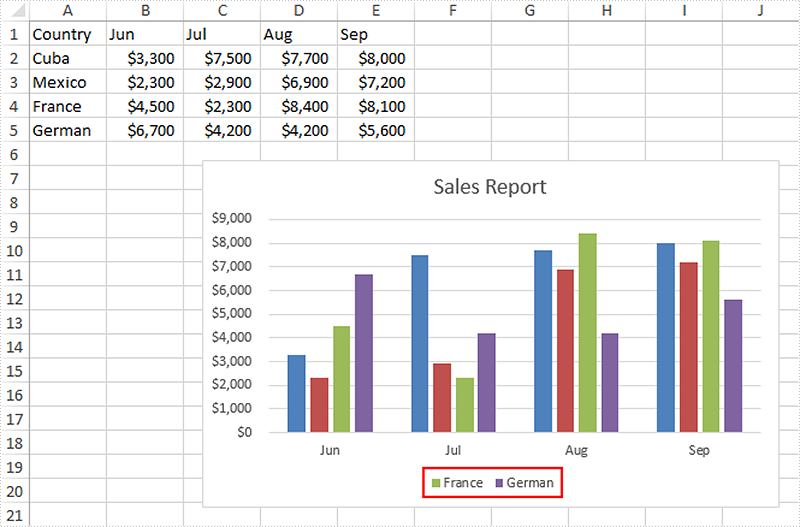Monday, 12 March 2018 07:12

### Create Multi-Level Category Chart in Excel in C#, VB.NET

Multi-level category chart is a chart type that has both main category and subcategory labels. This type of chart is useful when you have figures for items that belong to different categories. In this article, you will learn how to create a multi-level category chart in Excel using Spire.XLS with C# and VB.NET.

Step 1: Create a Workbook instance and get the first worksheet.

```Workbook wb = new Workbook();
Worksheet sheet = wb.Worksheets;
```

Step 2: Write data to cells.

```sheet.Range["A1"].Text = "Main Category";
sheet.Range["A2"].Text = "Fruit";
sheet.Range["A6"].Text = "Vegies";
sheet.Range["B1"].Text = "Sub Category";
sheet.Range["B2"].Text = "Bananas";
sheet.Range["B3"].Text = "Oranges";
sheet.Range["B4"].Text = "Pears";
sheet.Range["B5"].Text = "Grapes";
sheet.Range["B6"].Text = "Carrots";
sheet.Range["B7"].Text = "Potatoes";
sheet.Range["B8"].Text = "Celery";
sheet.Range["B9"].Text = "Onions";
sheet.Range["C1"].Text = "Value";
sheet.Range["C2"].Value = "52";
sheet.Range["C3"].Value = "65";
sheet.Range["C4"].Value = "50";
sheet.Range["C5"].Value = "45";
sheet.Range["C6"].Value = "64";
sheet.Range["C7"].Value = "62";
sheet.Range["C8"].Value = "89";
sheet.Range["C9"].Value = "57";
```

Step 3: Vertically merge cells from A2 to A5, A6 to A9.

```sheet.Range["A2:A5"].Merge();
sheet.Range["A6:A9"].Merge();
```

Step 4: Add a clustered bar chart to worksheet.

```Chart chart = sheet.Charts.Add(ExcelChartType.BarClustered);
chart.ChartTitle = "Value";
chart.PlotArea.Fill.FillType = ShapeFillType.NoFill;
chart.Legend.Delete();
```

Step 5: Set the data source of series data.

```chart.DataRange = sheet.Range["C2:C9"];
chart.SeriesDataFromRange = false;
```

Step 6: Set the data source of category labels.

```ChartSerie serie = chart.Series;
serie.CategoryLabels = sheet.Range["A2:B9"];
```

Step 7: Show multi-level category labels.

```chart.PrimaryCategoryAxis.MultiLevelLable = true;
```

Step 8: Save the file.

```wb.SaveToFile("output.xlsx", ExcelVersion.Version2013);
```

Output: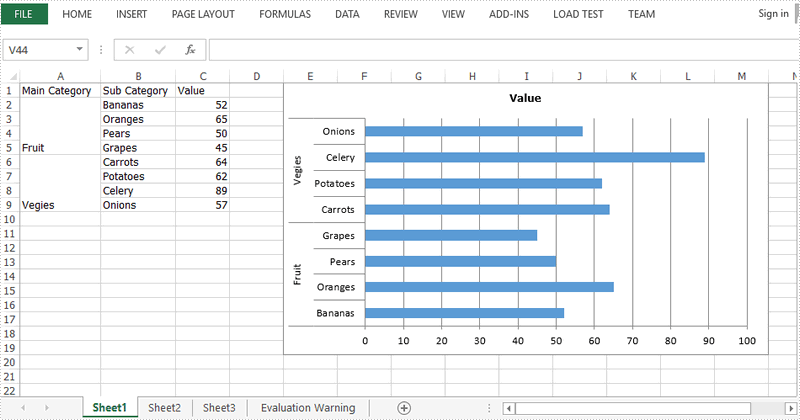Full Code:

[C#]
```Workbook wb = new Workbook();
Worksheet sheet = wb.Worksheets;

sheet.Range["A1"].Text = "Main Category";
sheet.Range["A2"].Text = "Fruit";
sheet.Range["A6"].Text = "Vegies";
sheet.Range["B1"].Text = "Sub Category";
sheet.Range["B2"].Text = "Bananas";
sheet.Range["B3"].Text = "Oranges";
sheet.Range["B4"].Text = "Pears";
sheet.Range["B5"].Text = "Grapes";
sheet.Range["B6"].Text = "Carrots";
sheet.Range["B7"].Text = "Potatoes";
sheet.Range["B8"].Text = "Celery";
sheet.Range["B9"].Text = "Onions";
sheet.Range["C1"].Text = "Value";
sheet.Range["C2"].Value = "52";
sheet.Range["C3"].Value = "65";
sheet.Range["C4"].Value = "50";
sheet.Range["C5"].Value = "45";
sheet.Range["C6"].Value = "64";
sheet.Range["C7"].Value = "62";
sheet.Range["C8"].Value = "89";
sheet.Range["C9"].Value = "57";
sheet.Range["A2:A5"].Merge();
sheet.Range["A6:A9"].Merge();
sheet.AutoFitColumn(1);
sheet.AutoFitColumn(2);

chart.ChartTitle = "Value";
chart.PlotArea.Fill.FillType = ShapeFillType.NoFill;
chart.Legend.Delete();
chart.LeftColumn = 5;
chart.TopRow = 1;
chart.RightColumn = 14;
chart.DataRange = sheet.Range["C2:C9"];
chart.SeriesDataFromRange = false;
ChartSerie serie = chart.Series;
serie.CategoryLabels = sheet.Range["A2:B9"];
chart.PrimaryCategoryAxis.MultiLevelLable = true;
wb.SaveToFile("output.xlsx", ExcelVersion.Version2013);
```
[VB.NET]
```Dim wb As Workbook = New Workbook()
Dim sheet As Worksheet = wb.Worksheets(0)

sheet.Range("A1").Text = "Main Category"
sheet.Range("A2").Text = "Fruit"
sheet.Range("A6").Text = "Vegies"
sheet.Range("B1").Text = "Sub Category"
sheet.Range("B2").Text = "Bananas"
sheet.Range("B3").Text = "Oranges"
sheet.Range("B4").Text = "Pears"
sheet.Range("B5").Text = "Grapes"
sheet.Range("B6").Text = "Carrots"
sheet.Range("B7").Text = "Potatoes"
sheet.Range("B8").Text = "Celery"
sheet.Range("B9").Text = "Onions"
sheet.Range("C1").Text = "Value"
sheet.Range("C2").Value = "52"
sheet.Range("C3").Value = "65"
sheet.Range("C4").Value = "50"
sheet.Range("C5").Value = "45"
sheet.Range("C6").Value = "64"
sheet.Range("C7").Value = "62"
sheet.Range("C8").Value = "89"
sheet.Range("C9").Value = "57"
sheet.Range("A2:A5").Merge()
sheet.Range("A6:A9").Merge()
sheet.AutoFitColumn(1)
sheet.AutoFitColumn(2)

Dim chart As Chart = sheet.Charts.Add(ExcelChartType.BarClustered)
chart.ChartTitle = "Value"
chart.PlotArea.Fill.FillType = ShapeFillType.NoFill
chart.Legend.Delete()
chart.LeftColumn = 5
chart.TopRow = 1
chart.RightColumn = 14
chart.DataRange = sheet.Range("C2:C9")
chart.SeriesDataFromRange = False
Dim serie As ChartSerie =  chart.Series(0)
serie.CategoryLabels = sheet.Range("A2:B9")
chart.PrimaryCategoryAxis.MultiLevelLable = True
wb.SaveToFile("output.xlsx", ExcelVersion.Version2013)
```
Thursday, 08 March 2018 03:46

### How to clone a word document in C#

With Spire.Doc, we can copy the content from one word document to another word document in C#. When we need to generate a large amount of documents from a single document, clone the document will be much easier. The clone method speeds up the generation of the word documents and developers only need one single line of code to get the copy of the word document.

Now we will show the code snippet of how to clone a word document in C#.

Step 1: Create a new instance of Document and load the document from file.

```Document doc = new Document();
```

Step 2: Clone the word document.

```doc.Clone();
```

Step 3: Save the document to file.

```doc.SaveToFile("Cloneword.docx", FileFormat.Docx2010);
```

Effective screenshot of clone the word document: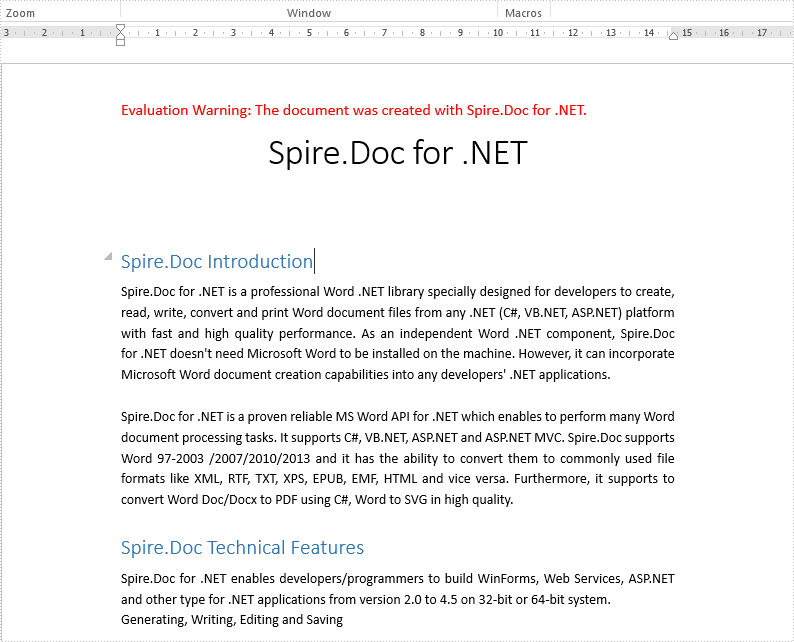Full codes of clone a word document:

```static void Main(string[] args)
{

Document doc = new Document();

doc.Clone();

doc.SaveToFile("Cloneword.docx", FileFormat.Docx2010);

}
```
Wednesday, 07 March 2018 07:51

### Delete Chart Legend Entries in PowerPoint in C#

When creating chart, the legend appears by default. If we just need the chart series rather than the legend, we can delete the legend entries after creating the chart. Spire.Presentation supports to delete legend entries from chart by using ChartLegend.DeleteEntry method. This article is going to demonstrate how we can use Spire.Presentation to accomplish this function.

Below screenshot shows the example PowerPoint file which contains 3 chart legend entries: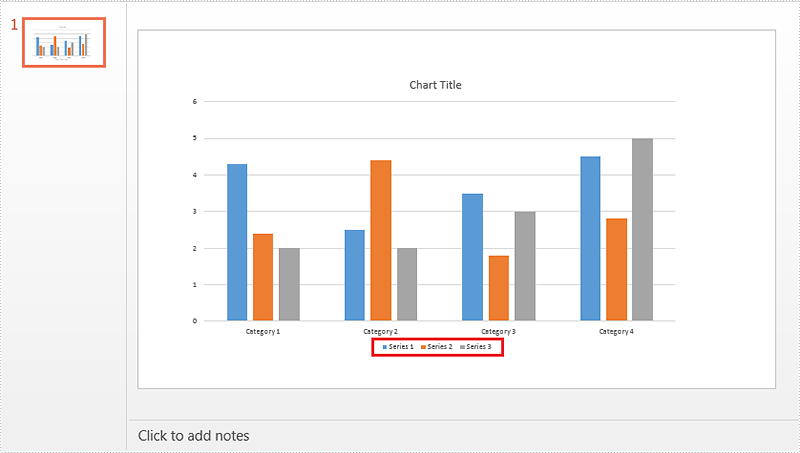Detail steps:

Step 1: Initialize an object of Presentation class and load the PowerPoint file.

```Presentation ppt = new Presentation();
```

Step 2: Get the chart.

```IChart chart = ppt.Slides.Shapes as IChart;
```

Step 3: Delete the first and the second legend entries from the chart.

```chart.ChartLegend.DeleteEntry(0);
chart.ChartLegend.DeleteEntry(1);
```

Step 4: Save the file.

```ppt.SaveToFile("Output.pptx", FileFormat.Pptx2010);
```

Screenshot: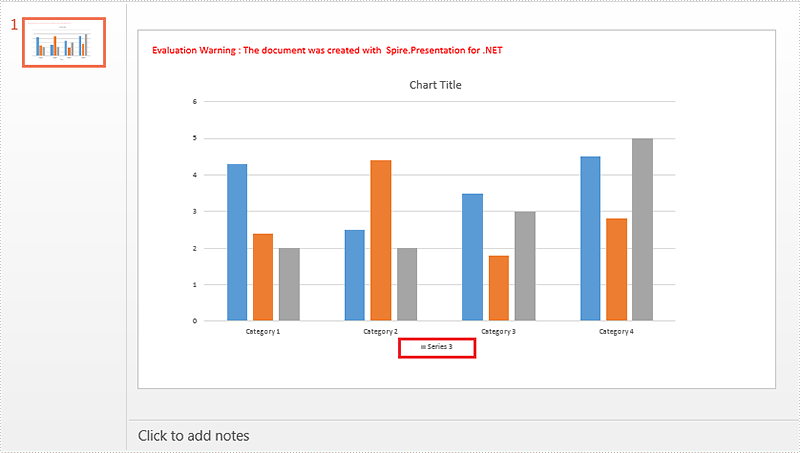Full code:

```using Spire.Presentation;
using Spire.Presentation.Charts;

namespace Delete_chart_legend_entries_in_PPT
{
class Program
{
static void Main(string[] args)
{
Presentation ppt = new Presentation();

IChart chart = ppt.Slides.Shapes as IChart;

chart.ChartLegend.DeleteEntry(0);
chart.ChartLegend.DeleteEntry(1);

ppt.SaveToFile("Output.pptx", FileFormat.Pptx2010);
}
}
}
```
Wednesday, 07 March 2018 06:41

### Group Two-Level Axis Labels in a Chart in PowerPoint in C#, VB.NET

Sometimes, you may have a chart that contains two levels of horizontal axis labels, as shown in the following screenshot, and you need to group the labels by fruit and vegies. This article will show you how to group the category axis labels using Spire.Presentation.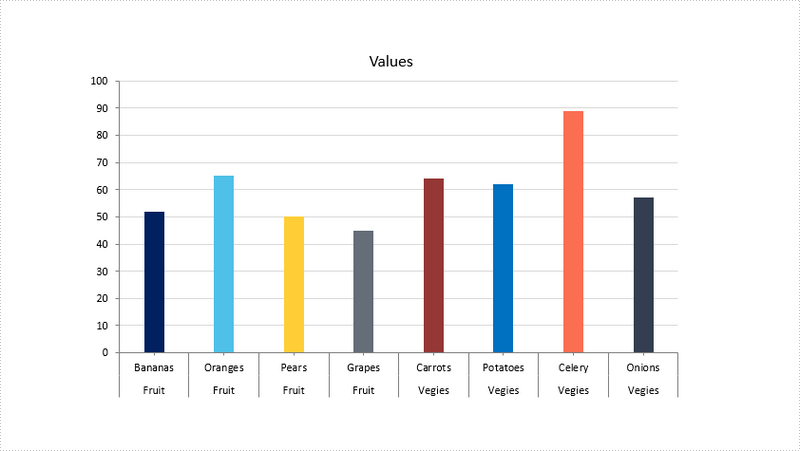Step 1: Create a Presentation instance and load the sample PowerPoint file.

```Presentation ppt = new Presentation();
```

Step 2: Get the chart.

```IChart chart = ppt.Slides.Shapes as IChart;
```

Step 3: Get the category axis from the chart.

```IChartAxis chartAxis = chart.PrimaryCategoryAxis;
```

Step 4: Determine if the axis has multilevel labels, if yes, group the axis labels that have the same first-level label.

```if(chartAxis.HasMultiLvlLbl)
{
chartAxis.IsMergeSameLabel = true;
}
```

Step 5: Save the file.

```ppt.SaveToFile("result.pptx", FileFormat.Pptx2010);
```

Output: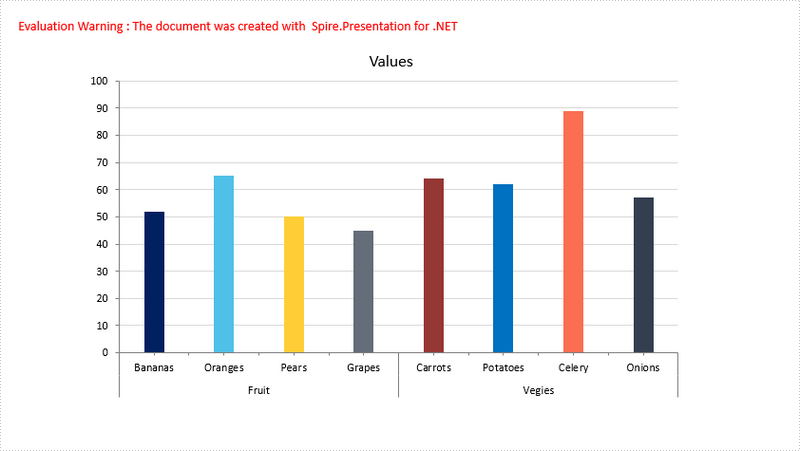Full Code:

[C#]
```Presentation ppt = new Presentation();
IChart chart = ppt.Slides.Shapes as IChart;
IChartAxis chartAxis = chart.PrimaryCategoryAxis;
if(chartAxis.HasMultiLvlLbl)
{
//group labels
chartAxis.IsMergeSameLabel = true;
}
ppt.SaveToFile("result.pptx", FileFormat.Pptx2010);
```
[VB.NET]
```Dim ppt As Presentation = New Presentation()